Home MATHEMATICS TOPIC 2: FRACTIONS ~ MATHEMATICS FORM 1

# TOPIC 2: FRACTIONS ~ MATHEMATICS FORM 1

55
0
SHAREA fraction is a number which is expressed in the form of a/b where a – is the top number called numerator and b– is the bottom number called denominator.

Proper, Improper and Mixed Numbers

A Fraction

Describe a fraction
A fraction is a number which is expressed in the form of a/b where a – is the top number called numerator and b– is the bottom number called denominator.
Consider the diagram below
The shaded part in the diagram above is 1 out of 8, hence mathematically it is written as 1/8Example 1
(a) 3 out of 5 ( three-fifths) = 3/5
Example 2
(b) 7 0ut of 8 ( i.e seven-eighths) = 7/8
Example 3
1. 5/12=(5 X 3)/(12 x 3) =15/36
2. 3/8 =(3 x 2)/(8 X 2) = 6/16
Dividing the numerator and denominator by the same number (This method is used to simplify the fraction)
Difference between Proper, Improper Fractions and Mixed Numbers
Distinguish proper, improper fractions and mixed numbers
Proper fraction –is a fraction in which the numerator is less than denominator
Example 4
4/5, 1/2, 11/13
Improper fraction -is a fraction whose numerator is greater than the denominator
Example 5
12/7, 4/3, 65/56
Mixed fraction –is a fraction which consist of a whole number and a proper fraction
Example 6(a) To convert mixed fractions into improper fractions, use the formula below
(b)To convert improper fractions into mixed fractions, divide the numerator by the denominatorExample 7
Convert the following mixed numbers into improper fractionsIn order to find which fraction is greater than the other, put them over a common denominator, and then the greater fraction is the one with greater numerator.
A Fraction to its Lowest Terms
Simplify a fraction to its lowest terms
Example 8
For the pair of fractions below, find which is greater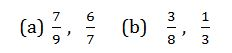Solution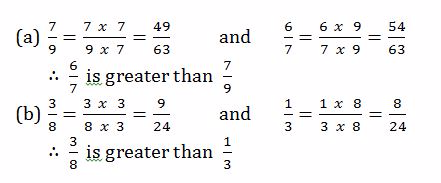Equivalent Fractions
Identify equivalent fractions
Equivalent Fraction
• Are equal fractions written with different denominators
• They are obtained by two methods
(a) Multiplying the numerator and denominator by the same number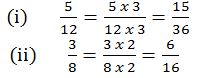(a) Dividing the numerator and denominator by the same number (This method is used to simplify the fractionNOTE: The fraction which cannot be simplified more is said to be in its lowest form
Example 9
Simplify the following fractions to their lowest terms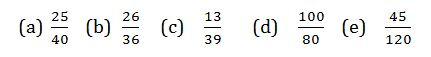Solution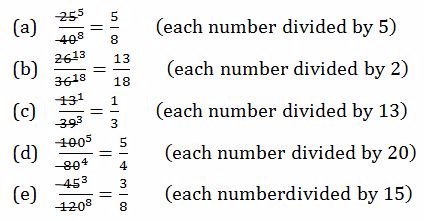Fractions in Order of Size
Arrange fractions in order of size
Example 10
Arrange in order of size, starting with the smallest, the fraction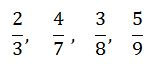Solution
Put them over the same denominator, that is find the L.C.M of 3, 7, 8 and 9
Operations on fractions involves addition, subtraction, multiplication and division
• Addition and subtraction of fractions is done by putting both fractions under the same denominator and then add or subtract
• Multiplication of fractions is done by multiplying the numerator of the first fraction with the numerator of the second fraction, and the denominator of the first fraction with the denominator the second fraction.
• For mixed fractions, convert them first into improper fractions and then multiply
• Division of fractions is done by taking the first fraction and then multiply with the reciprocal of the second fraction
• For mixed fractions, convert them first into improper fractions and then divide
Example 11
Find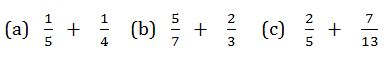Solution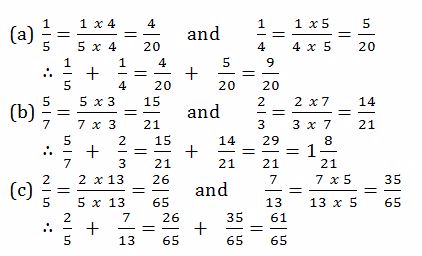Subtraction of Fractions
Subtract fractions
Example 12
Evaluate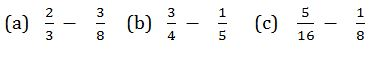Solution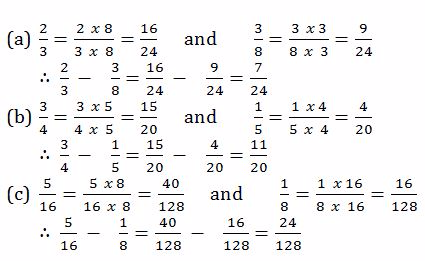Multiplication of Fractions
Multiply fractions
Example 13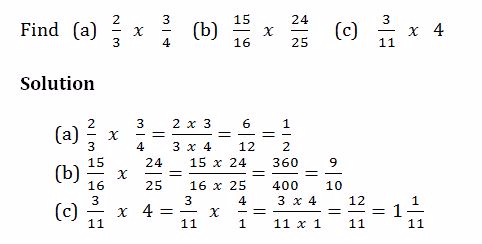Division of Fractions
Divide fractions
Example 14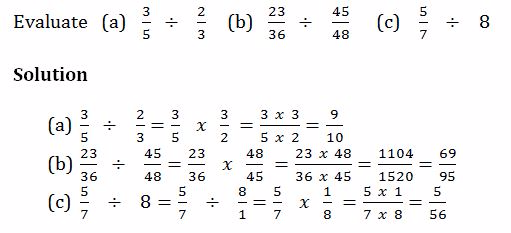Mixed Operations on Fractions
Perform mixed operations on fractions
Example 15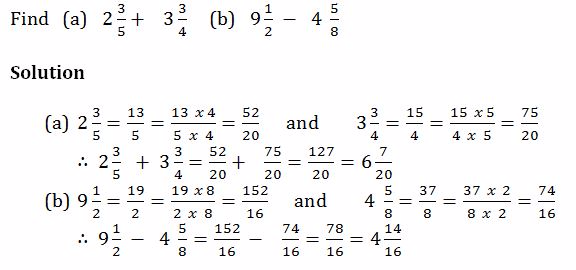Example 16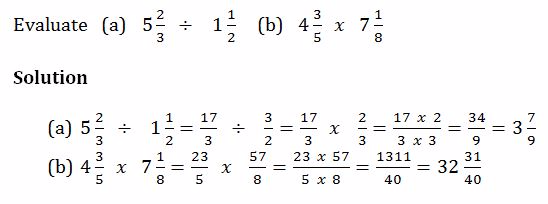Word Problems Involving Fractions
Solve word problems involving fractions
Example 17
1. Musa is years old. His father is 3¾times as old as he is. How old is his father?
2. 1¾of a material are needed to make suit. How many suits can be made from
SHARE
Previous articleTOPIC 1: NUMBERS ~ MATHEMATICS FORM 1